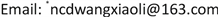﻿ 一类非线性时滞微分系统的稳定性 The Stabilities for a Class of Nonlinear Differential Systems with Time-Delay

Vol. 08  No. 11 ( 2019 ), Article ID: 33111 , 7 pages
10.12677/AAM.2019.811214

The Stabilities for a Class of Nonlinear Differential Systems with Time-Delay

Ran Huo1, Xiaoli Wang2*

1College of Science, Inner Mongolia Agriculture University, Huhhot Inner Mongolia

2College of Statistics and Mathematics, Inner Mongolia University Financial and Economics, Huhhot Inner MongoliaReceived: Oct. 31st, 2019; accepted: Nov. 15th, 2019; published: Nov. 22nd, 2019ABSTRACT

In this paper, we discuss the stability for a class of nonlinear differential systems by using integral inequalities.

Keywords:Integral Inequalities, Monotonous, Time-Delay Nonlinear Differential System, Stability

1内蒙古农业大学理学院数学与统计学系，内蒙古 呼和浩特

2内蒙古财经大学统计与数学学院数学系，内蒙古 呼和浩特1. 准备工作

$x\left(t\right)=\phi \left(t\right)$$\stackrel{˙}{x}\left(t\right)=\stackrel{˙}{\phi }\left(t\right)$ $\forall t\in {E}_{{t}_{0}}=\left[{t}_{0}-\tau ,{t}_{0}\right]$ 连续可微 (1.2)

(I)：常数 $c>0$

(II)： $\begin{array}{c}u\left(t\right)\le c+{\int }_{0}^{t}a\left(s\right)u\left(s\right)\text{d}s+\underset{i=1}{\overset{I}{\sum }}{\int }_{0}^{t}{b}_{i}\left(s\right){u}^{{\alpha }_{i}+1}\left(s\right)\text{d}s\\ \text{\hspace{0.17em}}\text{\hspace{0.17em}}+\underset{j=1}{\overset{J}{\sum }}{\int }_{0}^{t}{c}_{j}\left(s\right){\int }_{0}^{s}{d}_{j}\left(\sigma \right)u\left(\sigma \right)\text{d}\sigma \text{d}s\\ \text{\hspace{0.17em}}\text{\hspace{0.17em}}+\underset{k=1}{\overset{K}{\sum }}{\int }_{0}^{t}{e}_{k}\left(s\right){\int }_{0}^{s}{f}_{k}\left(\sigma \right){u}^{{\beta }_{k}+1}\left(\sigma \right)\text{d}\sigma \text{d}s\\ \text{\hspace{0.17em}}\text{\hspace{0.17em}}+{\int }_{0}^{t}\underset{i=1}{\overset{I}{\sum }}{b}_{i}\left(s\right){u}^{{\alpha }_{i}}\left(s\right)\left[\underset{l=1}{\overset{L}{\sum }}{\int }_{0}^{s}{g}_{l}\left(\sigma \right){\int }_{0}^{\sigma }{h}_{l}\left(\tau \right)u\left(\tau \right)\text{d}\tau \text{d}\sigma \right]\text{d}s\\ \text{\hspace{0.17em}}\text{\hspace{0.17em}}+{\int }_{0}^{t}\underset{i=1}{\overset{I}{\sum }}{b}_{i}\left(s\right){u}^{{\alpha }_{i}}\left(s\right)\left[\underset{m=1}{\overset{M}{\sum }}{\int }_{0}^{s}{p}_{m}\left(\sigma \right){\int }_{0}^{\sigma }{q}_{m}\left(\tau \right){u}^{{\gamma }_{m}+1}\left(\tau \right)\text{d}\tau \text{d}\sigma \right]\text{d}s\end{array}$

${e}_{k}\left(t\right),{f}_{k}\left(t\right)\text{\hspace{0.17em}}\left(k=1,2,\cdots ,K\right)$${g}_{l}\left(t\right),{h}_{l}\left(t\right)\text{\hspace{0.17em}}\left(l=1,2,\cdots ,L\right)$

${p}_{m}\left(t\right),{q}_{m}\left(t\right)\text{\hspace{0.17em}}\left(m=1,2,\cdots ,M\right)$ 均为 ${R}_{+}$ 上非负连续函数，

(III) 设 $\begin{array}{c}A\left(t\right)=a\left(t\right)+\underset{i=1}{\overset{I}{\sum }}{b}_{i}\left(t\right)+\underset{j=1}{\overset{J}{\sum }}{c}_{j}\left(t\right){\int }_{0}^{t}{d}_{j}\left(s\right)\text{d}s+2\underset{k=1}{\overset{K}{\sum }}{e}_{k}\left(t\right){\int }_{0}^{t}{f}_{k}\left(s\right)\text{d}s\\ \text{\hspace{0.17em}}\text{\hspace{0.17em}}+\underset{i=1}{\overset{I}{\sum }}{b}_{i}\left(t\right){\int }_{0}^{t}\underset{l=1}{\overset{L}{\sum }}{g}_{l}\left(s\right){\int }_{0}^{s}{h}_{l}\left(\sigma \right)\text{d}\sigma \text{d}s+\underset{i=1}{\overset{I}{\sum }}{b}_{i}\left(t\right){\int }_{0}^{t}\underset{m=1}{\overset{M}{\sum }}{p}_{m}\left(s\right){\int }_{0}^{s}{q}_{m}\left(\sigma \right)\text{d}\sigma \text{d}s\end{array}$

$\begin{array}{c}B\left(t\right)=\underset{i=1}{\overset{I}{\sum }}{b}_{i}\left(t\right)+\underset{k=1}{\overset{K}{\sum }}{e}_{k}\left(t\right){\int }_{0}^{t}{f}_{k}\left(s\right)\text{d}s+\underset{i=1}{\overset{I}{\sum }}{b}_{i}\left(t\right){\int }_{0}^{t}\underset{l=1}{\overset{L}{\sum }}{g}_{l}\left(s\right){\int }_{0}^{s}{h}_{l}\left(\sigma \right)\text{d}\sigma \text{d}s\\ \text{\hspace{0.17em}}\text{\hspace{0.17em}}+2\underset{i=1}{\overset{I}{\sum }}{b}_{i}\left(t\right){\int }_{0}^{t}\underset{m=1}{\overset{M}{\sum }}{p}_{m}\left(s\right){\int }_{0}^{s}{q}_{m}\left(\sigma \right)\text{d}\sigma \text{d}s\end{array}$

$C\left(t\right)={\int }_{0}^{t}\underset{m=1}{\overset{M}{\sum }}{p}_{m}\left(s\right){\int }_{0}^{s}{q}_{m}\left(\sigma \right)\text{d}\sigma \text{d}s$

${E}_{1}\left(t\right)=1-\stackrel{¯}{\alpha }{c}^{\stackrel{¯}{\alpha }}{\int }_{0}^{t}\left[B\left(\tau \right)+C\left(\tau \right)\right]\mathrm{exp}\left(\stackrel{¯}{\alpha }{\int }_{0}^{\tau }A\left(\sigma \right)\text{d}\sigma \right)\text{d}\tau$

$F\left(t\right)=a\left(t\right)+\underset{j=1}{\overset{J}{\sum }}{c}_{j}\left(t\right){\int }_{0}^{t}{d}_{j}\left(s\right)\text{d}s$

$\begin{array}{c}G\left(t\right)=\underset{i=1}{\overset{I}{\sum }}{b}_{i}\left(t\right){Щ}^{{\alpha }_{i}-\underset{_}{\alpha }}+\underset{k=1}{\overset{K}{\sum }}{e}_{k}\left(t\right){\int }_{0}^{t}{f}_{k}\left(s\right){Щ}^{{\beta }_{k}-\underset{_}{\alpha }}\text{d}s\\ \text{\hspace{0.17em}}\text{\hspace{0.17em}}+\underset{i=1}{\overset{I}{\sum }}{b}_{i}\left(t\right){N}^{{\alpha }_{i}-\underset{_}{\alpha }}{\int }_{0}^{t}\underset{l=1}{\overset{L}{\sum }}{g}_{l}\left(s\right){\int }_{0}^{s}{h}_{l}\left(\sigma \right)\text{d}\sigma \end{array}$

$H\left(t\right)=\underset{m=1}{\overset{M}{\sum }}{p}_{m}\left(t\right){\int }_{0}^{t}{q}_{m}\left(s\right){Щ}^{{\gamma }_{m}-\underset{_}{\alpha }}\text{d}s$

${E}_{2}\left(t\right)=1-\underset{_}{\alpha }{c}^{\underset{_}{\alpha }}{\int }_{0}^{t}\left[G\left(\tau \right)+H\left(\tau \right)\right]\mathrm{exp}\left(\underset{_}{\alpha }{\int }_{0}^{\tau }F\left(\sigma \right)\text{d}\sigma \right)\text{d}\tau$

${\int }_{0}^{+\infty }A\left(s\right)\text{d}s<+\infty$

${E}_{1}\left(t\right)>0$$\left[1-\left(\stackrel{¯}{\alpha }-1\right){c}^{\stackrel{¯}{\alpha }}{\int }_{0}^{t}B\left(s\right){E}_{1}^{-\frac{1}{\stackrel{¯}{\alpha }}}\left(s\right)\mathrm{exp}\left(\stackrel{¯}{\alpha }{\int }_{0}^{s}A\left(\tau \right)\text{d}\tau \right)\text{d}s\right]>0$

${E}_{2}\left(t\right)>0$$\left[1-\left(\underset{_}{\alpha }-1\right){c}^{\underset{_}{\alpha }}{\int }_{0}^{t}G\left(s\right){E}_{2}^{-\frac{1}{\underset{_}{\alpha }}}\left(s\right)\mathrm{exp}\left(\underset{_}{\alpha }{\int }_{0}^{s}F\left(\tau \right)\text{d}\tau \right)\text{d}s\right]>0$

2. 一类非线性时滞微分系统的稳定性

$\frac{\text{d}x}{\text{d}t}=A\left(t\right)x\left(t\right)+f\left(t,x\left(t-\delta \left(t\right)\right),{\int }_{0}^{t}h\left\{s,x\left(s-\delta \left(s\right)\right),{\int }_{0}^{s}g\left[\sigma ,x\left(\sigma -\delta \left(\sigma \right)\right)\right]\text{d}\sigma \right\}\text{d}s\right)$ (2.1)

$h\in C\left[I×{R}^{n}×{R}^{n}×{R}^{n},{R}^{n}\right]$$g\in C\left[I×{R}^{n}×{R}^{n},{R}^{n}\right]$

$0<{\delta }_{0}\le \delta \left(t\right)\le \delta$$A\left(t\right)$ 有界， $f\left(t,0,0,0\right)=0$$x\left(t\right)=\phi \left(t\right)$，于 $-\delta \le t\le 0$

$\begin{array}{c}x\left(t\right)=Y\left(t,0\right)\phi \left(0\right)\\ +{\int }_{0}^{t}Y\left(t,s\right)f\left(s,x\left(s-\delta \left(s\right)\right),{\int }_{0}^{s}h\left\{\tau ,x\left(\tau -\delta \left(\tau \right)\right),{\int }_{0}^{\tau }g\left[\sigma ,x\left(\sigma -\delta \left(\sigma \right)\right)\right]\text{d}\sigma \right\}\text{d}\tau \right)\text{d}s\end{array}$ (2.2)

1) $‖Y\left(t,s\right)‖\le \mathrm{exp}\left(r\left(t-s\right)\right)$$\left(t\ge s\ge 0\right)$$r\le 0$

2) $\begin{array}{l}‖f\left(t,x\left(t-\delta \left(t\right)\right),{\int }_{0}^{t}h\left\{s,x\left(s-\delta \left(s\right)\right),{\int }_{0}^{s}g\left[\sigma ,x\left(\sigma -\delta \left(\sigma \right)\right)\right]\text{d}\sigma \right\}\text{d}s\right)‖\\ \le a\left(t\right)‖x\left(t-\delta \left(t\right)\right)‖+\underset{i=1}{\overset{I}{\sum }}{b}_{i}\left(t\right){‖x\left(t-\delta \left(t\right)\right)‖}^{{\alpha }_{i}+1}\\ \text{\hspace{0.17em}}\text{\hspace{0.17em}}+\underset{j=1}{\overset{J}{\sum }}{c}_{j}\left(t\right){\int }_{0}^{t}\mathrm{exp}\left(r\left(t-s\right)\right){d}_{j}\left(s\right)‖x\left(s-\delta \left(s\right)\right)‖\text{d}s\\ \text{\hspace{0.17em}}\text{\hspace{0.17em}}+\underset{k=1}{\overset{K}{\sum }}{e}_{k}\left(t\right){\int }_{0}^{t}\mathrm{exp}\left(r\left(t-s\right)\right){f}_{k}\left(s\right){‖x\left(s-\delta \left(s\right)\right)‖}^{{\beta }_{k}+1}\text{d}s\\ \text{\hspace{0.17em}}\text{\hspace{0.17em}}+\underset{i=1}{\overset{I}{\sum }}{b}_{i}\left(t\right){‖x\left(t-\delta \left(t\right)\right)‖}^{{\alpha }_{i}}{\int }_{0}^{t}\mathrm{exp}\left(r\left(t-s\right)\right)\underset{l=1}{\overset{L}{\sum }}{g}_{l}\left(s\right){\int }_{0}^{s}{h}_{l}\left(\sigma \right)‖x\left(\sigma -\delta \left(\sigma \right)\right)‖\text{d}\sigma \text{d}s\\ \text{\hspace{0.17em}}\text{\hspace{0.17em}}+\underset{i=1}{\overset{I}{\sum }}{b}_{i}\left(t\right){‖x\left(t-\delta \left(t\right)\right)‖}^{{\alpha }_{i}}{\int }_{0}^{t}\underset{m=1}{\overset{M}{\sum }}\mathrm{exp}\left(r\left(t-s\right)\right){p}_{m}\left(s\right){\int }_{0}^{s}{q}_{m}\left(\sigma \right){‖x\left(\sigma -\delta \left(\sigma \right)\right)‖}^{{\gamma }_{m}+1}\text{d}\sigma \text{d}s\end{array}$

3) 其中 $a\left(t\right)$${b}_{i}\left(t\right)\text{\hspace{0.17em}}\left(i=1,2,\cdots ,I\right)$${c}_{j}\left(t\right),{d}_{j}\left(t\right)\text{\hspace{0.17em}}\left(j=1,2,\cdots ,J\right)$

${e}_{k}\left(t\right),{f}_{k}\left(t\right)\text{\hspace{0.17em}}\left(k=1,2,\cdots ,K\right)$${g}_{l}\left(t\right),{h}_{l}\left(t\right)\text{\hspace{0.17em}}\left(l=1,2,\cdots ,L\right)$

${p}_{m}\left(t\right),{q}_{m}\left(t\right)\text{\hspace{0.17em}}\left(m=1,2,\cdots ,M\right)$ 如  中定理 3.1.1 所设，且单调不增。

4) $F\left(t\right)={M}_{2}a\left(t\right)+{M}_{2}\underset{j=1}{\overset{J}{\sum }}{c}_{j}\left(t\right){\int }_{0}^{t}{d}_{j}\left(t\right)\text{d}t$${\int }_{0}^{+\infty }F\left(s\right)\text{d}s<+\infty$

$\begin{array}{c}G\left(t\right)=\underset{i=1}{\overset{I}{\sum }}{M}_{2}{b}_{i}\left(t\right){\text{e}}^{{\alpha }_{i}rt}{Щ}^{{\alpha }_{i}-\underset{_}{\alpha }}+\underset{k=1}{\overset{K}{\sum }}{M}_{2}{e}_{k}\left(t\right){\int }_{0}^{t}{f}_{k}\left(s\right){\text{e}}^{{\beta }_{k}rs}{Щ}^{{\beta }_{k}-\underset{_}{\alpha }}\text{d}s\\ \text{\hspace{0.17em}}\text{\hspace{0.17em}}+\underset{i=1}{\overset{I}{\sum }}{M}_{2}{b}_{i}\left(t\right){\text{e}}^{{\alpha }_{i}rt}{N}^{{\alpha }_{i}-\underset{_}{\alpha }}{\int }_{0}^{t}\underset{l=1}{\overset{L}{\sum }}{g}_{l}\left(s\right){\int }_{0}^{s}{h}_{l}\left(\sigma \right)\text{d}\sigma \end{array}$

$H\left(t\right)=\underset{m=1}{\overset{M}{\sum }}{M}_{2}{p}_{m}\left(t\right){\int }_{0}^{t}{q}_{m}\left(s\right){\text{e}}^{{\gamma }_{m}rs}{Щ}^{{\gamma }_{m}-\underset{_}{\alpha }}\text{d}s$

$E\left(t\right)=1-\underset{_}{\alpha }{c}^{\underset{_}{\alpha }}{\int }_{0}^{t}\left[G\left(\tau \right)+H\left(\tau \right)\right]\mathrm{exp}\left(\underset{_}{\alpha }{\int }_{0}^{\tau }F\left(\sigma \right)\text{d}\sigma \right)\text{d}\tau$

$E\left(t\right)>0$，且， $\left[1-\left(\underset{_}{\alpha }-1\right){c}^{\underset{_}{\alpha }}{\int }_{0}^{t}G\left(s\right){E}^{-\frac{1}{\underset{_}{\alpha }}}\left(s\right)\mathrm{exp}\left(\underset{_}{\alpha }{\int }_{0}^{s}F\left(\tau \right)\text{d}\tau \right)\text{d}s\right]>0$

${\int }_{0}^{\infty }G\left(s\right){E}^{-\frac{1}{\underset{_}{\alpha }}}\left(s\right)\mathrm{exp}\left(\underset{_}{\alpha }{\int }_{0}^{s}F\left(\tau \right)\text{d}\tau \right)\text{d}s<\infty$ 其中 $Щ$ 如    中引理所设

$x\left(t\right)=Y\left(t,0\right)\phi \left(0\right)+{\int }_{0}^{t}Y\left(t,s\right)f\left(s,x\left(s-\delta \left(s\right)\right),{\int }_{0}^{s}h\left\{\tau ,x\left(\tau -\delta \left(\tau \right)\right),{\int }_{0}^{\tau }g\left[\sigma ,x\left(\sigma -\delta \left(\sigma \right)\right)\right]\text{d}\sigma \right\}\text{d}\tau \right)\text{d}s$

$\begin{array}{c}‖x\left(t\right)‖\le ‖\phi \left(0\right)‖\mathrm{exp}\left(rt\right)+{\int }_{0}^{t}\mathrm{exp}\left(r\left(t-s\right)\right)a\left(s\right)‖x\left(s-\delta \left(s\right)\right)‖\text{d}s\\ \text{\hspace{0.17em}}\text{\hspace{0.17em}}+{\int }_{0}^{t}\underset{i=1}{\overset{I}{\sum }}{b}_{i}\left(s\right)\mathrm{exp}\left(r\left(t-s\right)\right){‖x\left(s-\delta \left(s\right)\right)‖}^{{\alpha }_{i}+1}\text{d}s\\ \text{\hspace{0.17em}}\text{\hspace{0.17em}}+{\int }_{0}^{t}\underset{j=1}{\overset{J}{\sum }}{c}_{j}\left(s\right)\mathrm{exp}\left(r\left(t-s\right)\right){\int }_{0}^{s}\mathrm{exp}\left(r\left(s-\theta \right)\right){d}_{j}\left(\theta \right)‖x\left(\theta -\delta \left(\theta \right)\right)‖\text{d}\theta \text{d}s\\ \text{\hspace{0.17em}}\text{\hspace{0.17em}}+{\int }_{0}^{t}\underset{k=1}{\overset{K}{\sum }}{e}_{k}\left(s\right)\mathrm{exp}\left(r\left(t-s\right)\right){\int }_{0}^{s}\mathrm{exp}\left(r\left(s-\theta \right)\right){f}_{k}\left(\theta \right){‖x\left(\theta -\delta \left(\theta \right)\right)‖}^{{\beta }_{k}+1}\text{d}\theta \text{d}s\end{array}$

$\begin{array}{l}\text{ }+{\int }_{0}^{t}\underset{i=1}{\overset{I}{\sum }}{b}_{i}\left(s\right){‖x\left(s-\delta \left(s\right)\right)‖}^{{\alpha }_{i}}\mathrm{exp}\left(r\left(t-s\right)\right)\\ \text{ }×{\int }_{0}^{s}\mathrm{exp}\left(r\left(s-\theta \right)\right)\underset{l=1}{\overset{L}{\sum }}{g}_{l}\left(\theta \right){\int }_{0}^{\theta }{h}_{l}\left(\sigma \right)‖x\left(\sigma -\delta \left(\sigma \right)\right)‖\text{d}\sigma \text{d}\theta \text{d}s\\ \text{ }+{\int }_{0}^{t}\underset{i=1}{\overset{I}{\sum }}{b}_{i}\left(s\right){‖x\left(s-\delta \left(s\right)\right)‖}^{{\alpha }_{i}}\mathrm{exp}\left(r\left(t-s\right)\right)\\ \text{ }×{\int }_{0}^{s}\underset{m=1}{\overset{M}{\sum }}\mathrm{exp}\left(r\left(s-\theta \right)\right){p}_{m}\left(\theta \right){\int }_{0}^{\theta }{q}_{m}\left(\sigma \right){‖x\left(\sigma -\delta \left(\sigma \right)\right)‖}^{{\gamma }_{m}+1}\text{d}\sigma \text{d}\theta \text{d}s\end{array}$ (2.3)

$\begin{array}{c}‖x\left(t\right)‖{\text{e}}^{-rt}\le ‖\phi \left(0\right)‖{\int }_{0}^{t}a\left(s\right)‖x\left(s-\delta \left(s\right)\right)‖{\text{e}}^{-rs}\text{d}s+{\int }_{0}^{t}\underset{i=1}{\overset{I}{\sum }}{b}_{i}\left(s\right){‖x\left(s-\delta \left(s\right)\right)‖}^{{\alpha }_{i}+1}{\text{e}}^{-rs}\text{d}s\\ \text{\hspace{0.17em}}\text{\hspace{0.17em}}+{\int }_{0}^{t}\underset{j=1}{\overset{J}{\sum }}{c}_{j}\left(s\right){\text{e}}^{-rs}{\int }_{0}^{s}{\text{e}}^{r\left(s-\theta \right)}{d}_{j}\left(\theta \right)‖x\left(\theta -\delta \left(\theta \right)\right)‖\text{d}\theta \text{d}s\\ \text{\hspace{0.17em}}\text{\hspace{0.17em}}+{\int }_{0}^{t}\underset{k=1}{\overset{K}{\sum }}{e}_{k}\left(s\right){\text{e}}^{-rs}{\int }_{0}^{s}{\text{e}}^{r\left(s-\theta \right)}{f}_{k}\left(\theta \right){‖x\left(\theta -\delta \left(\theta \right)\right)‖}^{{\beta }_{k}+1}\text{d}\theta \text{d}s\\ \text{\hspace{0.17em}}\text{\hspace{0.17em}}+{\int }_{0}^{t}\underset{i=1}{\overset{I}{\sum }}{b}_{i}\left(s\right){‖x\left(s-\delta \left(s\right)\right)‖}^{{\alpha }_{i}}{\text{e}}^{-rs}{\int }_{0}^{s}{\text{e}}^{r\left(s-\theta \right)}\underset{l=1}{\overset{L}{\sum }}{g}_{l}\left(\theta \right){\int }_{0}^{\theta }{h}_{l}\left(\sigma \right)‖x\left(\sigma -\delta \left(\sigma \right)\right)‖\text{ }\text{ }\text{d}\sigma \text{d}\theta \text{d}s\\ \text{\hspace{0.17em}}\text{\hspace{0.17em}}+{\int }_{0}^{t}\underset{i=1}{\overset{I}{\sum }}{b}_{i}\left(s\right){‖x\left(s-\delta \left(s\right)\right)‖}^{{\alpha }_{i}}{\text{e}}^{-rs}{\int }_{0}^{s}{\text{e}}^{r\left(s-\theta \right)}\underset{m=1}{\overset{M}{\sum }}{p}_{m}\left(\theta \right){\int }_{0}^{\theta }{q}_{m}\left(\sigma \right){‖x\left(\sigma -\delta \left(\sigma \right)\right)‖}^{{\gamma }_{m}+1}\text{d}\sigma \text{d}\theta \text{d}s\end{array}$ (2.4)

$u\left(t\right)=‖x\left(t\right)‖{\text{e}}^{-rt}$，由 ${\text{e}}^{-rt}={\text{e}}^{-r\left(t-\delta \left(t\right)\right)}\cdot {\text{e}}^{-r\delta \left(t\right)}$，注意到 $\delta \left(t\right)\le \delta$，故可设 $\underset{0\le t<+\infty }{\mathrm{sup}}{\text{e}}^{-r\delta \left(t\right)}={M}_{1}<+\infty$，由此可得：

$\begin{array}{c}u\left(t\right)\le ‖\phi \left(0\right)‖+{M}_{1}{\int }_{0}^{t}a\left(s\right)u\left(s-\delta \left(s\right)\right)\text{d}s+{\int }_{0}^{t}\underset{i=1}{\overset{I}{\sum }}{M}_{1}^{{\alpha }_{i}+1}{b}_{i}\left(s\right){\text{e}}^{{\alpha }_{i}rs}{u}^{{\alpha }_{i}+1}\left(s-\delta \left(s\right)\right)\text{d}s\\ \text{\hspace{0.17em}}\text{\hspace{0.17em}}+{M}_{1}{\int }_{0}^{t}\underset{j=1}{\overset{J}{\sum }}{c}_{j}\left(s\right){\int }_{0}^{s}{d}_{j}\left(\theta \right)u\left(\theta -\delta \left(\theta \right)\right)\text{d}\theta \text{ }\text{d}s\\ \text{\hspace{0.17em}}\text{\hspace{0.17em}}+{\int }_{0}^{t}\underset{k=1}{\overset{K}{\sum }}{M}_{1}^{{\beta }_{k}+1}{e}_{k}\left(s\right){\int }_{0}^{s}{\text{e}}^{{\beta }_{k}r\theta }{f}_{k}\left(\theta \right){u}^{{\beta }_{k}+1}\left(\theta -\delta \left(\theta \right)\right)\text{d}\theta \text{ }\text{d}s\\ \text{\hspace{0.17em}}\text{\hspace{0.17em}}+{\int }_{0}^{t}\underset{i=1}{\overset{I}{\sum }}{M}_{1}^{{\alpha }_{i}+1}{b}_{i}\left(s\right){u}^{{\alpha }_{i}}\left(s-\delta \left(s\right)\right){\text{e}}^{{\alpha }_{i}rs}{\int }_{0}^{s}\underset{l=1}{\overset{L}{\sum }}{g}_{l}\left(\theta \right){\int }_{0}^{\theta }{h}_{l}\left(\sigma \right)u\left(\sigma -\delta \left(\sigma \right)\right)\text{ }\text{ }\text{d}\sigma \text{d}\theta \text{d}s\\ \text{\hspace{0.17em}}\text{\hspace{0.17em}}+{\int }_{0}^{t}\underset{i=1}{\overset{I}{\sum }}{M}_{1}^{{\alpha }_{i}+1}{b}_{i}\left(s\right){u}^{{\alpha }_{i}}\left(s-\delta \left(s\right)\right){\text{e}}^{{\alpha }_{i}rs}{\int }_{\text{\hspace{0.17em}}0}^{s}\underset{m=1}{\overset{M}{\sum }}{p}_{m}\left(\theta \right){\int }_{0}^{\theta }{M}_{1}^{{\gamma }_{m}}{\text{e}}^{{\gamma }_{m}r\sigma }{q}_{m}\left(\sigma \right){u}^{{\gamma }_{m}+1}\left(\sigma -\delta \left(\sigma \right)\right)\text{ }\text{ }\text{d}\sigma \text{d}\theta \text{d}s\end{array}$ (2.5)

${\phi }_{3}=\underset{-\delta \le t\le 0}{\mathrm{sup}}{‖\phi \left(t\right)‖}^{{\beta }_{k}+1}$${\phi }_{4}=\underset{-\delta \le t\le 0}{\mathrm{sup}}{‖\phi \left(t\right)‖}^{{\gamma }_{m}+1}$

$\phi =\mathrm{max}\left\{{\phi }_{1},{\phi }_{2},{\phi }_{3},{\phi }_{4},{\phi }_{2}{\phi }_{4}\right\}$

$\begin{array}{c}u\left(t\right)\le \phi +{M}_{2}{\int }_{0}^{t}a\left(s\right)‖u\left(s\right)‖\text{d}s+{\int }_{0}^{t}\underset{i=1}{\overset{I}{\sum }}{M}_{2}{b}_{i}\left(s\right){\text{e}}^{{\alpha }_{i}rs}{‖u\left(s\right)‖}^{{\alpha }_{i}+1}\text{d}s\\ \text{\hspace{0.17em}}\text{\hspace{0.17em}}+{M}_{2}{\int }_{0}^{t}\underset{j=1}{\overset{J}{\sum }}{c}_{j}\left(s\right){\int }_{0}^{s}{d}_{j}\left(\theta \right)‖u\left(\theta \right)‖\text{d}\theta \text{d}s\\ \text{\hspace{0.17em}}\text{\hspace{0.17em}}+{\int }_{0}^{t}\underset{k=1}{\overset{K}{\sum }}{M}_{2}{e}_{k}\left(s\right){\int }_{0}^{s}{\text{e}}^{{\beta }_{k}r\theta }{f}_{k}\left(\theta \right){‖u\left(\theta \right)‖}^{{\beta }_{k}+1}\text{d}\theta \text{d}s\\ \text{\hspace{0.17em}}\text{\hspace{0.17em}}+{\int }_{0}^{t}\underset{i=1}{\overset{I}{\sum }}{M}_{2}{b}_{i}\left(s\right){‖u\left(s\right)‖}^{{\alpha }_{i}}{\text{e}}^{{\alpha }_{i}rs}{\int }_{0}^{s}\underset{l=1}{\overset{L}{\sum }}{g}_{l}\left(\theta \right){\int }_{0}^{\theta }{h}_{l}\left(\sigma \right)‖u\left(\sigma \right)‖\text{d}\sigma \text{d}\theta \text{d}s\\ \text{\hspace{0.17em}}\text{\hspace{0.17em}}+{\int }_{0}^{t}\underset{i=1}{\overset{I}{\sum }}{M}_{2}{b}_{i}\left(s\right){‖u\left(s\right)‖}^{{\alpha }_{i}}{\text{e}}^{{\alpha }_{i}rs}{\int }_{0}^{s}\underset{m=1}{\overset{M}{\sum }}{p}_{m}\left(\theta \right){\int }_{0}^{\theta }{\text{e}}^{{\gamma }_{m}r\sigma }{q}_{m}\left(\sigma \right){‖u\left(\sigma \right)‖}^{{\gamma }_{m}+1}\text{d}\sigma \text{d}\theta \text{d}s\end{array}$ (2.6)

$u\left(t\right)\le c\mathrm{exp}\left({\int }_{0}^{t}F\left(s\right)\text{d}s\right)\cdot {\left[1-\left(\underset{_}{\alpha }-1\right){c}^{\underset{_}{\alpha }}{\int }_{0}^{t}G\left(s\right){E}^{-\frac{1}{\underset{_}{\alpha }}}\left(s\right)\mathrm{exp}\left(\underset{_}{\alpha }{\int }_{0}^{s}F\left(\tau \right)\text{d}\tau \right)\text{d}s\right]}^{-\frac{1}{\underset{_}{\alpha }-1}}$

$‖x\left(t\right)‖\le \phi {\text{e}}^{rt}\mathrm{exp}\left({\int }_{0}^{t}F\left(s\right)\text{d}s\right){\left[1-\left(\underset{_}{\alpha }-1\right){c}^{\underset{_}{\alpha }}{\int }_{0}^{t}G\left(s\right){E}^{-\frac{1}{\underset{_}{\alpha }}}\left(s\right)\mathrm{exp}\left(\underset{_}{\alpha }{\int }_{0}^{s}F\left(\tau \right)\text{d}\tau \right)\text{d}s\right]}^{-\frac{1}{\underset{_}{\alpha }-1}}$

$r<0$${\int }_{0}^{+\infty }F\left(s\right)\text{d}s<+\infty$$E\left(t\right)>0$$\underset{_}{\alpha }\ge 1$

$\left[1-\left(\underset{_}{\alpha }-1\right){c}^{\underset{_}{\alpha }}{\int }_{0}^{t}G\left(s\right){E}^{-\frac{1}{\underset{_}{\alpha }}}\left(s\right)\mathrm{exp}\left(\underset{_}{\alpha }{\int }_{0}^{s}F\left(\tau \right)\text{d}\tau \right)\text{d}s\right]>0$，且有：

$\left[1-\left(\underset{_}{\alpha }-1\right){c}^{\underset{_}{\alpha }}{\int }_{0}^{t}G\left(s\right){E}^{-\frac{1}{\underset{_}{\alpha }}}\left(s\right)\mathrm{exp}\left(\underset{_}{\alpha }{\int }_{0}^{s}F\left(\tau \right)\text{d}\tau \right)\text{d}s\right]<1$，于是令：

$\begin{array}{c}M\left(t,0\right)={\text{e}}^{rt}\mathrm{exp}\left({\int }_{0}^{t}F\left(s\right)\text{d}s\right){\left[1-\left(\underset{_}{\alpha }-1\right){c}^{\underset{_}{\alpha }}{\int }_{0}^{t}G\left(s\right){E}^{-\frac{1}{\underset{_}{\alpha }}}\left(s\right)\mathrm{exp}\left(\underset{_}{\alpha }{\int }_{0}^{s}F\left(\tau \right)\text{d}\tau \right)\text{d}s\right]}^{-\frac{1}{\underset{_}{\alpha }-1}}\\ \le {\text{e}}^{rt}\mathrm{exp}\left({\int }_{0}^{t}F\left(s\right)\text{d}s\right)\end{array}$

The Stabilities for a Class of Nonlinear Differential Systems with Time-Delay[J]. 应用数学进展, 2019, 08(11): 1845-1851. https://doi.org/10.12677/AAM.2019.811214

1. 1. 斯力更. 具有变量时滞的非线性中立型微分方程组的有界性和稳定性[J]. 数学学报, 1974(3): 197-204.

2. 2. 斯力更. 关于Lipschitz稳定性的注记(I) [J]. 内蒙古师范大学学报, 1994(4): 1-4.

3. 3. 斯力更. 关于Lipschitz稳定性的注记(II) [J]. 内蒙古师范大学学报, 1995(2): 1-4.

4. 4. 斯力更. 关于Lipschitz稳定性的注记(III) [J]. 内蒙古师范大学学报, 1996(2): 1-5.

5. 5. Huo, R., Wang, X.L. and Si, L.G. (2009) A Generalization of a Class of Integral Inequality. Journal of Inner Mongolia Agricultural University (Natural Science Edition), 30, 267-273.

6. 6. 霍冉, 张彩琴, 王晓丽, 斯力更. 基于一类积分不等式上的中立型微分系统的Lipschitz稳定性[J]. 数学的实践与认识, 2009, 39(9): 231-234.

7. 7. 斯力更, 王凤. 一类非线性微分系统关于部分变元的稳定性[J]. 内蒙古师范大学学报(自然科学版), 2006, 35(1): 1-6.

8. 8. uo, R.,Wang, X.L. and Si, L.G. (2008) Exponential Asymptotic Stability for Nonlinear Neutral Systems with Multiple Delay. Journal of Xuzhou Normal University, 26, 80-84.

9. 9. 霍冉. 几类时滞微分方程的稳定性[D]: [硕士学位论文]. 呼和浩特: 内蒙古师范大学, 2008.

10. NOTES

*通讯作者。